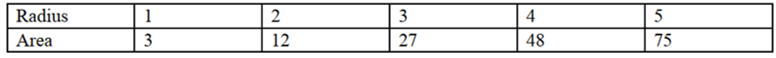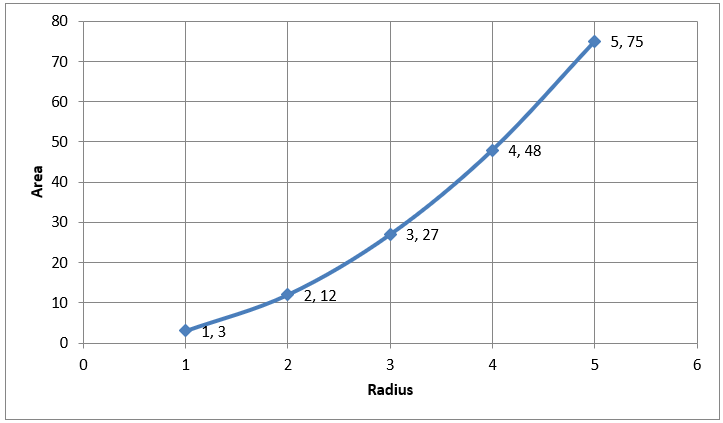Maths-
General
Easy

Question

# Meri Approximates the area of circles using the equation  and records areas of circles with different radius lengths in a table.a) Graph the ordered pairs from the tableb) Is the relation a function ? Explain.Hint:

## The correct answer is: graph is a function.

### Step by step solution:Let us denote the radius of the circle by x.Let us denote the area of the circle by y.Then the given table is x 1 2 3 4 5 y 3 12 27 48 75 We plot the above points in a graph.We can check if a graph is a function by the vertical line test. A graph represents a function if any vertical line in the xy plane cuts the graph at maximum one point. We use this method to check if the given graph represents a function.We can see that if we draw any vertical line in the xy plane, it cuts the graph at exactly one point.Hence, the given graph is a function.

In a function, every value of x must have one value of y. When we draw a vertical, it cuts x axis at one point, we take that point to be x. If the vertical line cuts the graph at two points, then it gives two values of y for one value of x. This does not represent a function.#### With Turito Foundation.#### Get an Expert Advice From Turito.